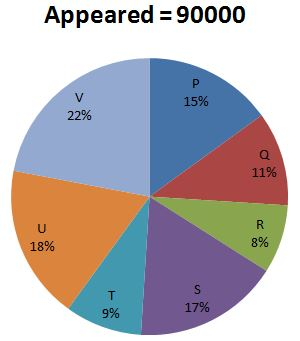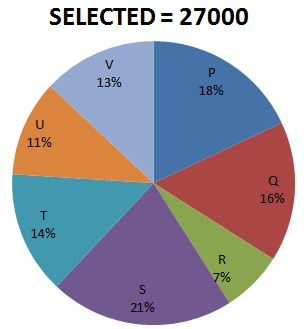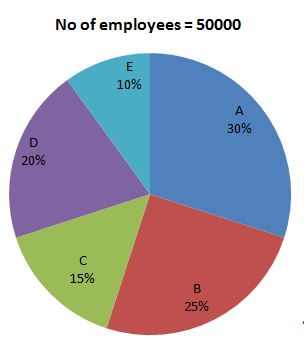#### D.1-5) The following pie chart 1 shows number of candidates appeared for different Posts. And the chart 2 shows number of candidates selected for the posts. By the two charts find the correct answer for the following questions.1) What is the ratio between number of candidates appeared for post R and T together and selected for the post S and V together?

a) 3 : 4

b) 4 : 5

c) 5 : 3

d) 4 : 7

e) 8 : 7

(C)

R & T appeared = 8+9=17%

17% of 90000=15300

Selected for S and V = 21+13 = 34%

34% of 27000 = 9180

Ratio = 15300:  9180

= 5:3

2) In which post qualified candidates to appeared candidate is maximum?

a) P

b) Q

c) R

d) S

e) T

(E)

P=  (27000×18)/(90000×15)×100=36%

Q=  (27000×16)/(90000×11)×100=43.63%

R=  (27000×7)/(90000×8)×100=26.25%

S=  (27000×21)/(90000×17)×100=37.05%

T=  (27000×14)/(90000×9)×100=46.67%

U=  (27000×11)/(90000×18)×100=18.33%

V=  (27000×13)/(90000×22)×100=17.72%

T is maximum

3) What is the difference between the number of appeared candidates for post Q and qualified candidate for post S?

a) 3600

b) 5670

c) 4230

d) 9900

e) 1234

(C)

Appeared for post Q = 90000×11/100=9900

Selected for post S = 27000×21/100=5670

Difference = 9900 – 5670

= 4230

4) What is the percentage of candidates selected to that of appeared candidates R and U together?

a) 20.77%

b) 31.66%

c) 27.65%

d) 23.5%

e) 18.67%

(A)

R and U appeared = 18 + 8% = 26%

90000×26/100=23400

R and U selected = 7 + 11% = 18%

27000×18/100=4860

% of selected=4860/23400×100

=20.77%

5) What is the ratio between number of candidates appeared for post S and qualified candidates T and U together?

a) 34 : 15

b) 25 : 37

c) 30 : 23

d) 17 : 25

e) 23 : 33

(A)

Appeared for post of S = 17% of 90000

Selected for post of T and U = 14% + 11% = 25%

25% of 27000

=17 ×  90000 : 25 × 27000

= 17 × 10: 25 × 3

=17 × 2  : 5 × 3

=34  :  15

#### D.6-10) The following pie chart shows number of employees in different companies. By the find the correct answer for the following questions.6) If 20%of the employees in companies A and 25% of employees in company C were insured, then what is the non-insured employee ratio in company A and C?

a) 32 : 17

b) 35 : 17

c) 30 : 19

d) 32 : 15

e) 16 : 19

(D)

Companies A non insured = 50000×30/100×80/100=12000

Companies C non insured = 50000×15/100×75/100=5625

Ratio

= 12000: 5625

= 4000: 1875

=800: 375

= 160: 75

= 32: 15

7) If there is 5000 employees in company C, and 4000 employees in company D are insured then what is the total number of non-insured employees in both companies together?

a) 7350

b) 8500

c) 3700

d) 4800

e) 5000

(B)

Company C and D together = 20 +15 = 35%

35% of 50000 = 17500

Non insured = 17500-9000 = 8500

8) In company E 200 new employees were recruited and 25% of them have insurance policy, and new ratio between insured and non- insured becomes 6 : 7 then what was the ratio of insured and uninsured before that employees joined in the company.

a) 5 : 6

b) 47 : 53

c) 37 : 43

d) 6 : 7

e) 38 : 45

(B)

Company E’s employees before recruitment = 50000×10/100=5000

New employees = 200

And insured and non insured = 50 and 150

New ratio = 6: 7 from 5200

= 2400: 2800

Uninsured before = 2800-150=2650

Insured before = 2400-50=2350

Ratio of company E’s before recruitment insured to non-insured= 2350: 2650

= 47: 53

9) 20% of A, 30% of B, 30% of C, 60% of D and 70% of E are getting salaries above Rs.10000 and 30% of employees getting salary above 10000 are getting above 20000 as their salary, then how many employees are getting salary above 20000.

a) 5550

b) 3750

c) 4850

d) 3000

e) 6500

(A)

A = 50000×30/100×20/100×30/100= 900

B = 50000×25/100×30/100×30/100= 1125

C = 50000×15/100×30/100×30/100= 675

D = 50000×20/100×60/100×30/100= 1800

E = 50000×10/100×70/100×30/100= 1050

=900 + 1125 + 675 + 1800 + 1050 = 5550

10) If 20% of employees in company A are in officer grade and 60% of are labour and remaining are company staffs. What is the difference between the employees in officer grade and company staffs?

a) 3000

b) 1500

c) 4500

d) 5000

e) No such difference.